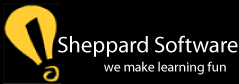Quick Math - Basic calculating - adding, subtracting, multiplying and dividing (mixed operations) are the fundamental building blocks to learning math! Use our Quick Math game to get kids excited at calculating faster and faster. The faster kids learn basic arithmetic and calculating, the easier a time they'll have with learning and figuring out algebra, SAT math and all other math!